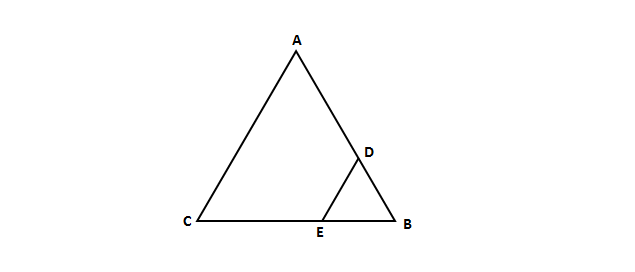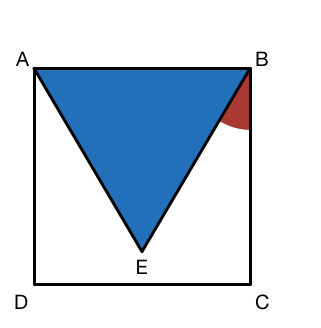Geometry

# Regular Polygons - Problem Solving$\triangle ABC$ is a regular triangle with side length $6.$ If a triangle $\triangle BDE$ is cut from $\triangle ABC$, where the length of $\overline{DB}$ and $\overline{EB}$ are both equal to $1$, what is the perimeter of remaining figure $ADEC?$

Which of the following facts about regular polygons is false?$ABCD$ is a square. The point $E$ is located within $ABCD$, such that $ABE$ is an equilateral triangle. What is the measure (in degrees) of $\color{#D61F06} {\angle \text{CBE} }$?

Consider a polygon with $n$ vertices such that all internal angles are obtuse. If the sum of $n-1$ angles in this polygon is $973^{\circ},$ what is the last angle in degrees?

A regular polygon has interior angles of $150^\circ$. $A, B, C, D$ are 4 consecutive points of this polygon. What is the measure (in degrees) of $\angle ADC?$

×